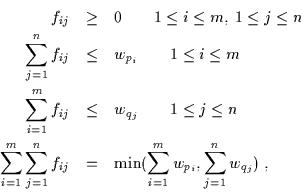## 1.常见的距离算法

1.1 欧几里得距离（Euclidean DistanceEuclidean Distance是一个通常采用的距离定义，它是在m维空间中两个点之间的真实距离。

>>> pdist = nn.PairwiseDistance(p=2)
>>> input1 = torch.randn(100, 128)
>>> input2 = torch.randn(100, 128)
>>> output = pdist(input1, input2)

1.2 Earth Mover's Distance (EMD距离）

EMD问题通俗解释: Earth Move翻译过来是搬土，指把P位置的m个坑的土，用最小的代价搬到Q位置的n个坑中，dij是pi到qj两个坑的距离，fij是从pi搬到qj的土量，则WORK工作量就是要最小化的目标。线性规划求解出fij后，再用fij对WORK作个归一化，就得到了EMD。EMD 实际上是线性规划中运输问题的最优解。

EMD具体定义可参考：
EMDhomepages.inf.ed.ac.ukC代码包： emd.h, emd.c, emd.i

OpenCV：实现了EMD api,
pip install numpy Matplotlib
pip install opencv-python

import numpy as np
import cv

#p、q是两个矩阵，第一列表示权值，后面三列表示直方图或数量
p=np.asarray([[0.4,100,40,22],
[0.3,211,20,2],
[0.2,32,190,150],
[0.1,2,100,100]],np.float32)
q=np.array([[0.5,0,0,0],
[0.3,50,100,80],
[0.2,255,255,255]],np.float32)
pp=cv.fromarray(p)
qq=cv.fromarray(q)
emd=cv.CalcEMD2(pp,qq,cv.CV_DIST_L2)

1.3 曼哈顿距离（Manhattan Distance）: 表示两个点在标准坐标系上的绝对轴距之和。也就是和象棋中的“車”一样横平竖直的走过的距离。曼哈顿距离是超凸度量。1.4 杰卡德距离（Jaccard Distance）: 用来衡量两个集合差异性的一种指标，它是杰卡德相似系数的补集，被定义为1减去Jaccard相似系数。适用于集合相似性度量，字符串相似性度量 。1.5 马氏距离（Mahalanobis distance）: 表示点与一个分布之间的距离。它是一种有效的计算两个未知样本集的相似度的方法。与欧氏距离不同的是，它考虑到各种特性之间的联系（例如：一条关于身高的信息会带来一条关于体重的信息，因为两者是有关联的），并且是尺度无关的(scale-invariant)，即独立于测量尺度。修正了欧式距离中各个维度尺度不一致且相关的问题。import numpy as np
def mashi_distance(x,y):
print x
print y
#马氏距离要求样本数要大于维数，否则无法求协方差矩阵
#此处进行转置，表示10个样本，每个样本2维
X=np.vstack([x,y])

print X
XT=X.T

print XT

#方法一：根据公式求解
S=np.cov(X)   #两个维度之间协方差矩阵
SI = np.linalg.inv(S) #协方差矩阵的逆矩阵
#马氏距离计算两个样本之间的距离，此处共有4个样本，两两组合，共有6个距离。
n=XT.shape
d1=[]
for i in range(0,n):
for j in range(i+1,n):
delta=XT[i]-XT[j]
d=np.sqrt(np.dot(np.dot(delta,SI),delta.T))
print d
d1.append(d)
1. 切比雪夫距离（Chebyshev Distance）
2. 明可夫斯基距离（Minkowski Distance）
3. 海明距离（Hamming distance）
4. 马哈拉诺比斯距离（Mahalanobis Distance）

## 2.常见的相似度（系数）算法

2.1 余弦相似度（Cosine Similarity）>>> input1 = torch.randn(100, 128)
>>> input2 = torch.randn(100, 128)
>>> cos = nn.CosineSimilarity(dim=1, eps=1e-6)
>>> output = cos(input1, input2)

2.2 皮尔森相关系数（Pearson Correlation Coefficient）: 用于度量两个变量X和Y之间的相关（线性相关），其值介于-1与1之间。import numpy as np
x=np.random.random(8)
y=np.random.random(8)

#方法一：根据公式求解
x_=x-np.mean(x)
y_=y-np.mean(y)
d1=np.dot(x_,y_)/(np.linalg.norm(x_)*np.linalg.norm(y_))

#方法二：根据numpy库求解
X=np.vstack([x,y])
d2=np.corrcoef(X)

2.3 KL散度（Kullback-Leibler Divergence）: 即相对熵；是衡量两个分布(P、Q)之间的距离；越小越相似。>>> torch.nn.functional.kl_div(input, target, size_average=None, reduce=None, reduction='mean')
>>> F.kl_div(q.log(),p,reduction='sum')
#函数中的 p q 位置相反(也就是想要计算D(p||q)，要写成kl_div（q.log（），p）的形式)，而且q要先取 log
#reduction 是选择对各部分结果做什么操作，

2.4 Jaccard相似系数（Jaccard Coefficient）: 主要用于计算符号度量或布尔值度量的样本间的相似度。两个集合A和B的交集元素在A，B的并集中所占的比例，称为两个集合的杰卡德相似系数，用符号J(A,B)表示。杰卡德系数值越大，样本的相似度越高。import numpy as np
from scipy.spatial.distance import pdist
x=np.random.random(8)>0.5
y=np.random.random(8)>0.5

x=np.asarray(x,np.int32)
y=np.asarray(y,np.int32)

#方法一：根据公式求解
up=np.double(np.bitwise_and((x != y),np.bitwise_or(x != 0, y != 0)).sum())
down=np.double(np.bitwise_or(x != 0, y != 0).sum())
d1=(up/down)

#方法二：根据scipy库求解
X=np.vstack([x,y])
d2=pdist(X,'jaccard')

2.5 Tanimoto系数（广义Jaccard相似系数）import numpy as np
def tanimoto_coefficient(p_vec, q_vec):
"""
This method implements the cosine tanimoto coefficient metric
:param p_vec: vector one
:param q_vec: vector two
:return: the tanimoto coefficient between vector one and two
"""
pq = np.dot(p_vec, q_vec)
p_square = np.linalg.norm(p_vec)
q_square = np.linalg.norm(q_vec)
return pq / (p_square + q_square - pq)

2.6 互信息(Mutual Information) ：是信息论里一种有用的信息度量，它可以看成是一个随机变量 中包含的关于另一个随机变量的信息量，或者说是一个随机变量由于已知另一个随机变量而减少的不肯定性。衡量随机变量之间相互依赖程度的度量。#标准化互信息
from sklearn import metrics
if __name__ == '__main__':
A = [1, 1, 1, 2, 3, 3]
B = [1, 2, 3, 1, 2, 3]
result_NMI=metrics.normalized_mutual_info_score(A, B)
print("result_NMI:",result_NMI)
1. 对数似然相似度/对数似然相似率
2. 互信息/信息增益，相对熵/KL散度
3. 信息检索--词频-逆文档频率（TF-IDF）
4. 词对相似度--点间互信息